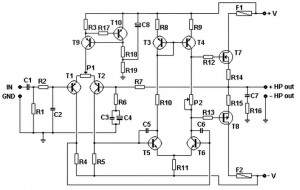# 100W Basic MOSFET amplifier – Schematic Diagrams

100W Basic MOSFET amplifier Schematic Diagrams      Comments Off on 100W Basic MOSFET amplifierThis Basic MOSFET amplifier is very simple to build and low cost. Is perfect for Hi-Fi amplifiers and instrument amplifiers (guitar, keyboards …). Output power is + / – 100 Wrms at 8 ohm load or + / – 160 Wrms below 4 ohms. The simplicity of this circuit cause distortion + / -0.1%. Bandwidth at -3 dB is from 4 Hz to 96 Khz and it is limited by C1, R1, R2 and C2.

Transistors T1 and T2 makes a first differential stage, current source of +/- 1 mA is set by R3. P1 allows a fine tuning of DC voltage at amplifier’s output. Place P1 at it’s half value for first power up, then turn it slowly for a lowest DC output voltage. It is recommended to use a first quality component.

Input sensitivity is 1.2 volts. The gain of 27x is archived by R7/R6. It may be modified by changing R7 value. Transistors T5 and T6 makes the second differential stage. Transistors T3 and T4 works as a current mirror source. They push the second differential stage to drain equal current. Doing so we get a high gain and an excellent linearity. Output MOSFET transistors works in AB class, their quiescient current is set from 50 to 100 mA trough P2. For setting quiescient current, you must set P2 in minimal resistance, place a multimeter in mV DC range accross on R14 or R15 leads, turn slowly the screw untill you read a 16,5 mV value, which correspond to a 50 mA quiescient current. F1 and F2 works as an elementary output short-circuit protection.The power supply must have a value between 45 and 55 Volts DC (positive and negative). One heatsink with a thermal resistance less of 2° C / W is required. All resistors are 1% metal film 1/4 watt.

Note :
Before connecting a speaker at amplifier output, connect a multimeter at output and look on DC output voltage. This level never may be greater than 50 mV. If it is so, check all amplifier for a mistake. Also, change T2 with another device and check again.

Parts list :C1 = 2.2 uF MKP, MKT 100 VC2 = 330 pF ceramic 50 VC3 = 100 nF MKP, MKT 100 VC4 = 40 uF 100 V electro-chemicalC5, C6 = 18 pF ceramic 50 VC7 = 100 nF MKP, MKT 250 VC8 = 47 uF 100 V)R1 = 47 KR 3 = 470 OhmsR2 = 2K2R4, R5 = 3K9R6 = 1 KR7 = 27 KR8, R9, R11 = 100 ohmsR10 = 10 KR12, R13 = 470 ohmsR14, R15 = 0.33 ohm 5 wattsR16 = 10 ohm 3 wattR17 = 1 KR18, R19 = 10KT1, T2, T9, T10 = 2N5401, ZTX558, BC556B (note the different pinout – take care for pin layout)T3, T4 = BF470, MJE350, 2SB649T5, T6 = BF469, MJE340, 2SD669T7 = IRFP240, 2SK1530, 2SJ162, BUZ900DP, BUZ901DP (note the different pinout – take care for pin layout: GDS GSD)T8 = IRFP9240, 2SJ201, 2SK1058, BUZ905DP, BUZ906DP (note the different pinout – take care for pin layout: GDS GSD)P1 = 100 ohms (25 laps – 25 turns)P2 = 2K5 (25 laps – 25 turns)F1, F2 = 3 T A     , , , ,